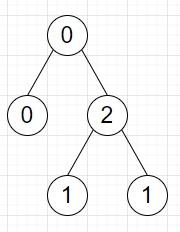# Program to count how many ways we can divide the tree into two trees in Python

Suppose we have a binary tree containing values 0, 1 and 2. The root has at least one 0 node and one 1 node. Now suppose there is an operation where we delete an edge in the tree and the tree becomes two different trees. We have to find the number of ways we can delete one edge such that none of the two trees contain both a 0 node and a 1 node.

So, if the input is likethen the output will be 1 as we can only delete the 0 to 2 edge.

To solve this, we will follow these steps −

• count := [0, 0, 0]
• Define a function dfs() . This will take node
• if node is not null, then
• pre := count
• dfs(left of node)
• dfs(right of node)
• count[value of node] := count[value of node] + 1
• node.count := a list of (count[i] - pre[i]) for i is 0 and 1
• Define a function dfs2() . This will take node, par
• if node is not null, then
• if par is not null, then
• (a0, a1) := count of node
• (b0, b1) := (count - a0, count - a1)
• if (a0 is same as 0 or a1 is same as 0) and (b0 is same as 0 or b1 is same as 0), then
• ans := ans + 1
• dfs2(left of node, node)
• dfs2(right of node, node)
• From the main method, do the following −
• dfs(root)
• ans := 0
• dfs2(root)
• return ans

Let us see the following implementation to get better understanding −

## Example

Live Demo

class TreeNode:
def __init__(self, data, left = None, right = None):
self.val = data
self.left = left
self.right = right
class Solution:
def solve(self, root):
count = [0, 0, 0]
def dfs(node):
if node:
pre = count[:]
dfs(node.left)
dfs(node.right)
count[node.val] += 1
node.count = [count[i] - pre[i] for i in range(2)]
dfs(root)
def dfs2(node, par=None):
if node:
if par is not None:
a0, a1 = node.count
b0, b1 = count - a0, count - a1
if (a0 == 0 or a1 == 0) and (b0 == 0 or b1 == 0):
self.ans += 1
dfs2(node.left, node)
dfs2(node.right, node)
self.ans = 0
dfs2(root)
return self.ans
ob = Solution()
root = TreeNode(0)
root.left = TreeNode(0)
root.right = TreeNode(2)
root.right.left = TreeNode(1)
root.right.right = TreeNode(1)
print(ob.solve(root))

## Input

root = TreeNode(0)
root.left = TreeNode(0)
root.right = TreeNode(2)
root.right.left = TreeNode(1)
root.right.right = TreeNode(1)

## Output

1